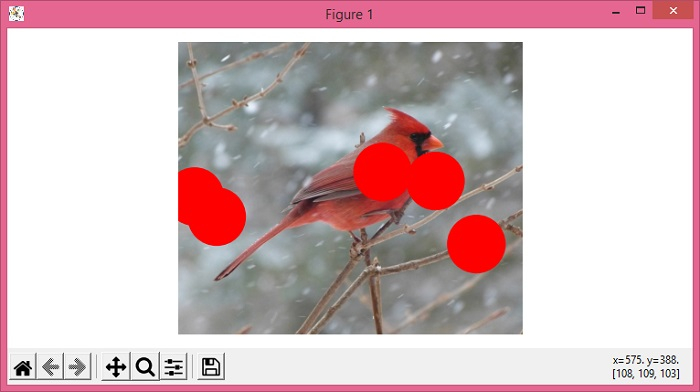# Drawing circles on an image with Matplotlib and NumPy

To draw a circle on an image with matplotlib and numpy, we can take the following steps −

• Set the figure size and adjust the padding between and around the subplots.

• Read an image from a file into an array.

• Create x and y data points using numpy.

• Create a figure and a set of subplots using subplots() method.

• Display data as an image, i.e., on a 2D regular raster using imshow() method.

• Turn off the axes.

• Add patches on the current axes.

• To display the figure, use show() method.

## Example

import matplotlib.pyplot as plt
import numpy as np
from matplotlib.patches import Circle

plt.rcParams["figure.figsize"] = [7.50, 3.50]
plt.rcParams["figure.autolayout"] = True

x = np.random.rand(5) * img.shape
y = np.random.rand(5) * img.shape

fig, ax = plt.subplots(1)
ax.imshow(img)
ax.axis('off')

for xx, yy in zip(x, y):
circ = Circle((xx, yy), 50, color='red')
plt.show()